# ECMAScript Loops Tutorial with Examples

## 1- Overview of the loops in ECMAScript

In ECMAScript, statements are executed sequentially from top to bottom. However, when you want to execute a sequence of statements multiple times, you can use loop.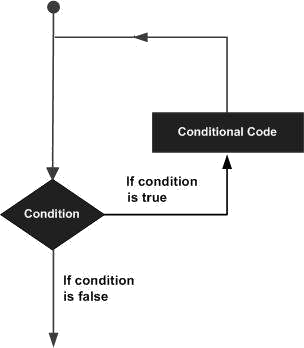ECMAScript provides you with 3 types of loops:
1. while loop
2. for loop
3. do .. while loop
Statements can be used within a loop:
1. continue
2. break
Control Statement Description

break

Terminates the loop statement.

continue

Causes the loop to skip the remainder of its body and immediately retest its condition prior to reiterating.

## 2- while loop

The syntax of a while loop:
``````
while (condition)  {
// Do something here
// ....
}
``````
Example:
while-loop-example.js
``````
console.log("While loop example");
// Declare a variable, and assign value of 2.
let x = 2;
// Condition is x < 10
// If x < 10 is true then run block
while (x < 10) {
console.log("Value of x = ", x);
x = x + 3;
}
// This statment is out of while block.
console.log("Finish");
``````
Output:
``````
While loop example
Value of x = 2
Value of x = 5
Value of x = 8
Finish
``````

## 3- for loop

The standard  for loop has the syntax as follows:
``````
for(InitialValues; condition; updateValues )
{
// Statements
}
``````
• InitialValues: Initialize  values for the related variables in the loop.
• condition: Defines the conditions for executing command blocks.
• updateValues: Update new values for variables
for-loop-example.js
``````
console.log("For loop example");
for( let i = 0; i < 10; i= i + 3)  {
console.log("i= "+ i);
}
``````
Output:
``````
For loop example
i= 0
i= 3
i= 6
i= 9
``````
for-loop-example2.js
``````
console.log("For loop example");
for(let i = 0, j = 0; i + j < 10; i = i+1, j = j+2)  {
console.log("i = " + i +", j = " + j);
}
``````
Output:
``````
For loop example
i = 0, j = 0
i = 1, j = 2
i = 2, j = 4
i = 3, j = 6
``````
Using the for loop can help you traverse on the elements of the array.
for-loop-example3.js
``````
console.log("For loop example");
// Array
let names =["Tom","Jerry", "Donald"];

for (let i = 0; i < names.length; i++ ) {
console.log("Name = ", names[i]);
}
console.log("End of example");
``````
Output:
``````
For loop example
Name = Tom
Name = Jerry
Name = Donald
End of example
``````

## 4- For .. in loop

The for..in loop helps you iterate over the properties of an object.
``````
for (prop in object) {
// Do something
}
``````
Example:
for-in-loop-example.js
``````
// An object has 4 properties (name, age, gender,greeing)
var myObject = {
name : "John",
age: 25,
gender: "Male",
greeting : function() {
return "Hello";
}
};
for(myProp in myObject)  {
console.log(myProp);
}
``````
Output:
``````
name
age
gender
greeting
``````

## 5- For .. of loop

The for..of loop helps you iterate over a Collection, for example, Map, Set.
Example:
for-of-example.js
``````
// Create a Set from an Array
var fruits = new Set( ["Apple","Banana","Papaya"] );

for(let fruit of fruits)  {
console.log(fruit);
}
``````
Output:
``````
Apple
Banana
Papaya
``````
for-of-example2.js
``````
// Create a Map object.
var myContacts = new Map();

myContacts.set("0100-1111", "Tom");
myContacts.set("0100-5555", "Jerry");
myContacts.set("0100-2222", "Donald");
for( let arr of myContacts)  {
console.log(arr);
console.log(" - Phone: " + arr);
console.log(" - Name: " + arr);
}
``````
Output:
``````
[ '0100-1111', 'Tom' ]
- Phone: 0100-1111
- Name: Tom
[ '0100-5555', 'Jerry' ]
- Phone: 0100-5555
- Name: Jerry
[ '0100-2222', 'Donald' ]
- Phone: 0100-2222
- Name: Donald
``````

## 6- do...while loop

The do-while loop is used to execute a section of program many times.The characteristics of the do-while is that the block of statement is always executed at least once. After each iteration, the program will check the condition, if the condition is still correct, the statement block will be executed again.
``````
do {
// Do something
}
while (expression);
``````
Example:
do-while-loop-example.js
``````
let value = 3;
do {
console.log("Value = " + value);
value = value + 3;
} while (value < 10);
``````
Output:
``````
Value = 3
Value = 6
Value = 9
``````

## 7- Use the break statement in the loop

break is a statement that may be located in a loop. This statement ends the loop unconditionally.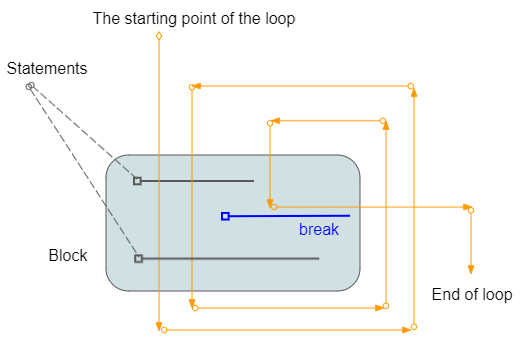loop-break-example.js
``````
console.log("Break example");
// Declare a variable and assign value of 2.
let x = 2;
while (x < 15) {
console.log("----------------------");
console.log("x = ", x);
// If x = 5 then exit the loop.
if (x == 5) {
break;
}
// Increase value of x by 1
x = x + 1;
console.log("x after + 1 = ", x);
}
console.log("End of example");
``````
Output:
``````
Break example
----------------------
x = 2
x after + 1 = 3
----------------------
x = 3
x after + 1 = 4
----------------------
x = 4
x after + 1 = 5
----------------------
x = 5
End of example
``````

## 8- Use the continue statement in the loop

continue is a statement which may be located in a loop. When caught the continue statement, the program will ignore the command lines in block, below of continue and start of a new loop.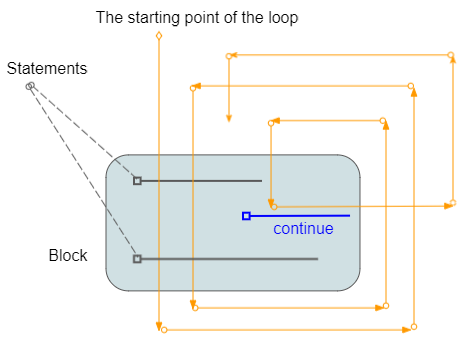loop-continue-example.js
``````
console.log("Continue example");
// Declare a variable and assign value of 2
x = 2
while (x < 7) {
console.log("----------------------")
console.log("x = ", x)
// % is used for calculating remainder
// If x is even, then ignore the command line below of continue
// and start new iteration.
if (x % 2 == 0) {
// Increase x by 1.
x = x + 1;
continue;
}
else {
// Increase x by 1.
x = x + 1
console.log("x after + 1 =", x)
}
}
console.log("End of example");
``````
Output:
``````
Continue example
----------------------
x = 2
----------------------
x = 3
x after + 1 = 4
----------------------
x = 4
----------------------
x = 5
x after + 1 = 6
----------------------
x = 6
End of example
``````

## 9- Labelled Loop

The ECMAScript allows you to stick a label to a loop. It is like you name a loop, which is useful when you use multiple nested loops in a program.
• You can use break labelX statement; to break a loop is attached labelX.
• You can use continue labelX statement; to continue a loop is attached​​​​​​​ labelX.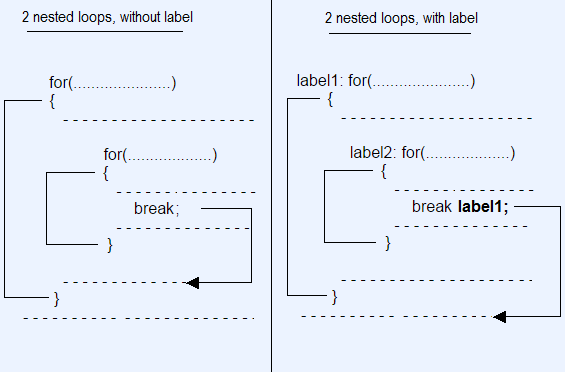Syntax:
``````
// for loop with Label.
label1: for( ... )  {

}
// while loop with Label.
label2: while ( ... )  {

}
// do-while loop with Label.
label3: do  {

} while ( ... );
``````
Example of using nested loops, labelled and labelled break statement.
labelled-loop-break-example.js
``````
console.log("Labelled Loop Break example");
let i = 0;
label1: while (i < 5) {
console.log("----------------------\n");
console.log("i = " + i);
i++;
label2: for (let j = 0; j < 3; j++) {
console.log("  --> " + j);
if (j > 0) {
// Exit the loop with label1.
break label1;
}
}
}
console.log("Done!");
``````
Output:
``````
Labelled Loop Break example
----------------------
i = 0
--> 0
--> 1
Done!
``````
Example of using nested labelled loops and labelled continue statement.
labelled-loop-continue-example.js
``````
let i = 0;
label1: while (i < 5) {
console.log("outer i= " + i);
i++;
label2: for (let j = 0; j < 3; j++) {
if (j > 0) {
continue label2;
}
if (i > 1) {
continue label1;
}
console.log("inner i= " + i + ", j= " + j);
}
}
``````
Output:
``````
outer i= 0
inner i= 1, j= 0
outer i= 1
outer i= 2
outer i= 3
outer i= 4
``````

#### View more Tutorials:

Maybe you are interested

These are online courses outside the o7planning website that we introduced, which may include free or discounted courses.

•The Complete JavaScript Bootcamp
•Getting really good at JavaScript and TypeScript
•Essentials of JavaScript Practice Coding Exercises Tips
•Learn JavaScript From Scratch:Become Top Rated Web Developer
•JavaScript For Beginners - Learn JavaScript From Scratch
•Javascript for Beginners
•Learning ECMAScript 6: Moving to the New JavaScript
•JavaScript Dynamic Quiz Application from Scratch JSON AJAX
•JavaScript Intro to learning JavaScript web programming
•Byte-Sized-Chunks: Dynamic Prototypes in Javascript
•Learn ECMAScript 2015 - ES6 Best Course
•Getting started with javascript and its core concepts
•Quick JavaScript Core learning Course JavaScript Essentials
•*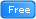* Master ECMAScript 2015 (ES6)
•Learn JavaScript Fundamentals
•HTML CSS JavaScript: Most popular ways to code HTML CSS JS
•** Start 3D GIS Web Development in JavaScript
•Learning JavaScript Programming Tutorial. A Definitive Guide
•2D Game Development With HTML5 Canvas, JS - Tic Tac Toe Game
•The Web Development Course: HTML5, CSS3, JavaScript
•JavaScript in 55 Minutes
•JavaScript for Beginning Web Developers
•JavaScript in Action JavaScript Projects
•The complete beginner JavaScript ES5, ES6 and JQuery Course
•** Introductory To JavaScript - Learn The Basics of JavaScript